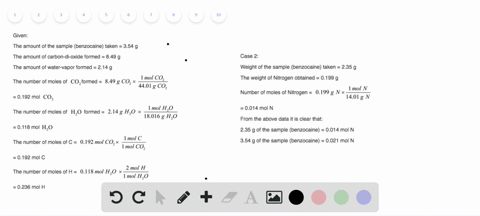### Researchers obtain the following data from experi…

01:51University of New Mexico
Problem 129

A compound of molar mass 177 g>mol contains only carbon, hydrogen, bromine, and oxygen. Analysis reveals that the compound contains 8 times as much carbon as hydrogen by mass. Find the molecular formula.

the molecular formula of the compound will be $\mathrm{C}_{6} \mathrm{H}_{9} \mathrm{BrO}$

## Discussion

You must be signed in to discuss.

## Video Transcript

the molar mass. Is this some off atomic masses off the atom? Present in a molecule, the mullahs moss off. The given compound is 117 grams. The compound only contains carbon hydrogen brew mean and oxygen. The compound contains eight thymus carbon as the hydrogen. So let consider the mass off my rusian be ex cramp. So the moss off carbon will be eight x graham. The atomic mass off bro mean and oxygen are given over here. So the total molar mass is 117. We can speculate the mass off hydrogen that this X, which turns out to be 90.1 gram per mole the second. But we need to deter mined the mood, more legal formula off the compound. So the number off moles off hydrogen turns out to be nine more as shown in the given calculation, we have the mass off carbon that is 72.8 crumbs so we can determine the moles off carbon. According to the givens, calculation is six months leading us to the final molecule of formula off the compound that this C six ej nine b R. Well,